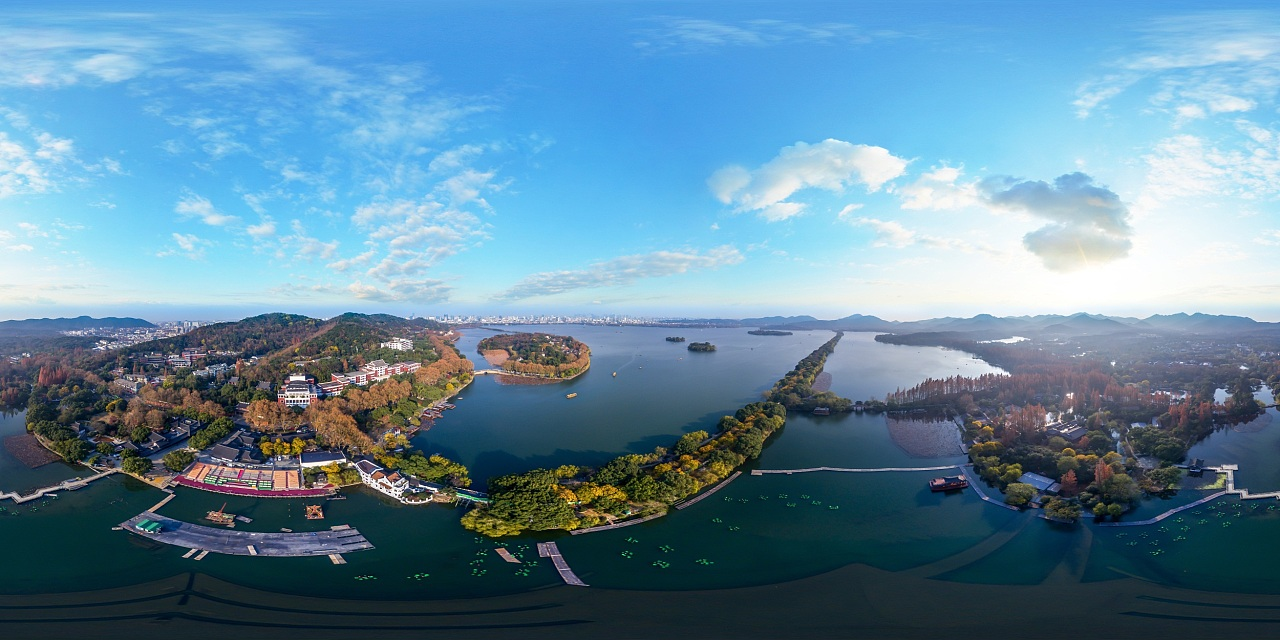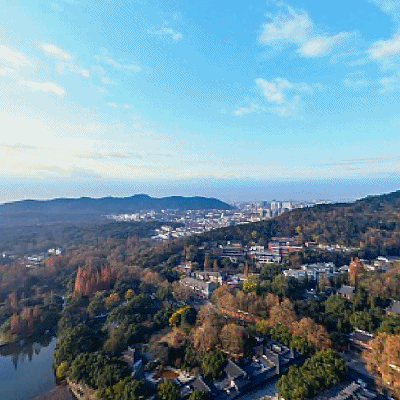1.  还是将全景图贴到球面，经纬展开法应该是老方法了。建立球面坐标系，x轴向右，y轴垂直向内，z轴向上，符合右手坐标系。

2. 仍然采用反向映射的方法，首先在球的南极点放置一张与南极点相切的平面（也就是我们要获取的局部平面图）。

3.设定视场角FOV，设定要获取的局部平面图的大小（W,H）。我们可以粗略计算出一个归一化的焦距f = (0.5*W)/tan(fov *0.5)。

4.局部视图上的坐标（u,v），转换到球面坐标系坐标为(x,y,z)：

其中x = u - W*0.5,    y = H*0.5 - v,     z =f

5. 得到(x, y, z)后就好办了，转换到球坐标（theta, fi）：

theta = acos( z/sqrt(x*x+y*y+z*z) )

fi = acos( x/sqrt(x*x+y*y) )   if (y<0)    fi=2*pi - fi

6.  得到球坐标后就可以获取全景图的坐标（U, V），如果全景图的尺寸是（panoW, panoH）：

U = fi * panoW/ (2 * pi);

V = theta * panoH / pi;

7. 老样子，双线性插值，从(U,V)周围四个点插值得到（u,v）。